## Calculating the price of a bond with an odd first period in SQL Server

Jul 29

Written by: Charles Flock
7/29/2014 10:32 AMWe have previously written about calculating price, yield, and accrued interest for bonds that have regular periodic coupons. However, not all bonds fit that description. Some bonds have an odd first period, meaning that the first coupon period is either shorter or longer than all the other coupons. In this article we will discuss how that affects the calculation of price, yield, and accrued interest and why you should not use the Excel functions for these types of calculations.
A bond has an odd first coupon (OFC) when the first coupon period is either longer or shorter than all the other coupon periods which then occur at regular intervals. In our previous article we discussed the math behind the calculation of price for bonds that pay regular periodic interest (RPI). For OFC bonds we need to make a slight adjustment to that formula in order to account for the odd first coupon, if the bond is settling before the first coupon date. If the bond settles on or after the first coupon date the price is calculated using the RPI formula.

The price equation
Here is the OFC price formula when the bond settles before the first coupon date.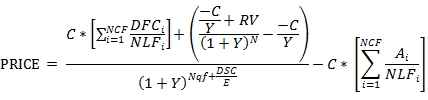Where

 Ai = number of accrued days for the ith quasi-coupon period C = 100 * coupon rate / frequency DFCi = number of days from the issue date to the first quasi-coupon date (i=1) or the number of days in the quasi-coupon period (i>1) DSC = number of days from settlement to coupon E = number of days in the quasi-coupon period N = number of coupons after the first coupon date through to the maturity date NCF = number of quasi-coupon periods that fit in the odd period NLFi = normal length in days of the full ith quasi-coupon period within the odd period. Nqf = the number of whole quasi-coupon period between the settlement date and the first coupon date RV = redemption value Y = yield / frequency

The first term in the numerator calculates the value of the first coupon; the amount of interest paid to the bond-holder on the first coupon date.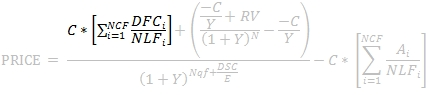In calculating the first coupon amount we can think of the first coupon period as consisting of one or more quasi-coupon periods. The quasi-coupon periods are the implied coupon dates, based on the maturity date, which occur before the first coupon date (which itself must be a regular coupon date with respect to the maturity date). We simply calculate the coupon dates until we get to a coupon date that is less than or equal to the issue date.
NCF identifies the number of quasi-coupon periods. While the formula contemplates that there could be more than 2 quasi-coupon periods, as a practical matter the number of quasi-coupon periods is either 1 or 2.
Having calculated the quasi-coupon dates, we simply multiply the regular coupon amount by the ratio of the days-to-first coupon to the normal length of the first coupon period; i.e. the number of days in the quasi-coupon period. The calculation of the NLF values is exactly the same as for any coupon period. The calculation of the DFC values, however, can be a little tricky. If the issue date falls in the quasi-coupon period, then the DFC value is the number of days in the coupon period (NLF) minus the number of days from the quasi-coupon date to the issue date. If the issue date does not fall in the quasi-coupon period then the DFC value is equal to the NLF value.
The second term in the numerator calculates the discounted cash flow value of all the remaining coupons and the redemption amount as of the first coupon date.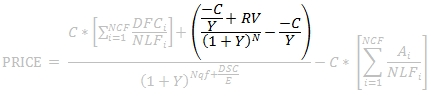N in this notation is different than N used in the PRICE function. In this case, N does not include the next (first) coupon date as it has already been accounted for in the first term.
The sum of these two terms provides the discounted value of the all the cash flows as of the first coupon date. The denominator simply discounts this value back to the settlement date.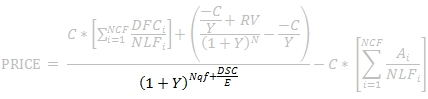DSC and E are calculated with respect to the quasi-coupon dates and are calculated in exactly the same way as in the PRICE function. Nqf is zero if there is only one quasi-coupon period, otherwise it is the number of whole quasi-coupon periods until the first coupon date. As a practical matter this means that if there are 2 quasi-coupon periods and the settlement date occurs in the first quasi-coupon period then Nqf is 1 otherwise Nqf is 0.
Finally, the accrued interest is subtracted from the discounted cash flow value.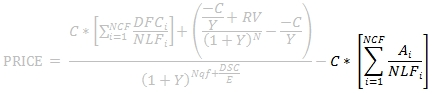The NLF values used in the calculation of the accrued interest are exactly the same values as the NLF values used in the calculation of the first coupon amount. A1 is the number of days from the quasi-start date to the settlement date minus the number of days from the quasi-start date to the issue date. If there are 2 quasi-coupon periods and the settlement date is greater than or equal to the quasi-coupon date, then A2 is the number of days from the quasi-coupon date to the settlement date.
Interestingly, if the coupon is zero, then the formula reduces to: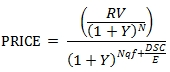which is equivalent to the formula for a bond that pays regular periodic interest. This equivalency is important to understand because it is not maintained in the Excel ODDFPRICE function.
Let's look at a very simple example of this, and see what the implications are in terms of the accuracy of the Excel ODDFPRICE function. Let's use the following bond as an example.

 Settlement: 2014-07-21 Maturity: 2034-11-30 Issue: 2014-02-15 First Coupon: 2014-11-30 Basis: Bond Coupon Rate: 2.35% Yield: 2.75% Redemption Value: 100 Coupon Frequency: 2

In XLeratorDB we can simply enter the following statement:
SELECT
wct.ODDFPRICE('2014-07-21','2034-11-30','2014-02-15','2014-11-30',.0235,.0275,100,2,0) as PRICE
This produces the following result.
PRICE
----------------------
93.7877040381472
When we enter the same data into the Excel ODDFPRICE formula, we get a price of 92.5018021749091. What causes that difference?
By changing the coupon rate to zero, we can analyze the cause of the problem in a very straightforward way. Just change .0235 to 0.0 in the SQL and in the Excel formula and you should get the following result.

 XLeratorDB Excel Zero-coupon price 57.3475483719767 56.5697147935652

As we pointed out above, the ODDFPRICE in the presence of a zero coupon rate should return the same result as the PRICE calculation. This SQL confirms that and returns the same result as the Excel PRICE function.
SELECT
wct.PRICE('2014-07-21','2034-11-30',0.0,.0275,100,2,0) as PRICE
This produces the following result.
PRICE
----------------------
57.3475483719767
We can use these zero-coupon prices to determine the number of coupon periods until the maturity date. The following SQL provides the answer:
SELECT
app
,zeroprice
,LOG(100/zeroprice)/LOG(1+.0275/2e+00) as [time-to-maturity]
FROM (VALUES
('XLeratorDB',57.3475483719767),('Excel',56.5697147935652)
)n(app,zeroprice)
This produces the following result.
app        zeroprice                               time-to-maturity
---------- --------------------------------------- ----------------------
XLeratorDB 57.3475483719767                        40.7166666666667
Excel      56.5697147935652                        41.7166666666666
It looks like the Excel calculation has one whole extra coupon period in its calculation.
In the following chart we compare the time-to-maturity calculation using the Excel ODDFPRICE and PRICE functions. This produces a very interesting chart, pictured below.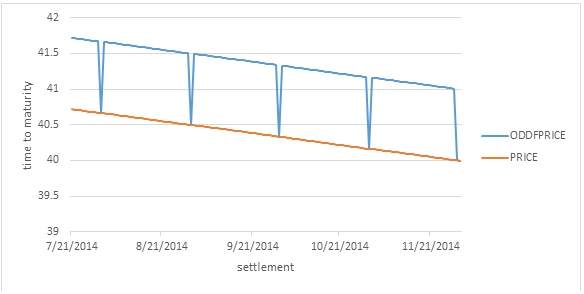As you can see, the difference between the Excel ODDFPRICE and PRICE calculation is exactly one coupon period from settlement date until the first coupon period, except that at 4 points the 2 lines intersect. These 4 points are the end of month dates for July, August, September, and October. The final point of intersection is the first coupon date, where the bond is no longer settling in the odd period.
We were pretty surprised at the unreliability of the Excel ODDFPRICE function. To help us in our analysis, we created the OFCFACTORS function which decomposes the values supplied to the ODDFPRICE function into the inputs into the equation. We can take our zero-coupon data and run the following SQL to see what those components are.
SELECT
*
FROM
wct.OFCFACTORS(
'2014-07-21'
,'2034-11-30'
,'2014-02-15'
,'2014-11-30'
,0.0
,NULL
,0.0275
,100
,2
,0)
This produces the following result (which is been reformatted to make it easier to read.

 E DSC N NCF A1 DFC1 NLF1 A2 DFC2 NLF2 Nqf 180 129 40 2 105 105 180 51 180 180 0

 quasistart quasicoup C FC P AI Y 2013-11-30 2014-05-31 0 0 57.34754837 0 0.0275

It is then very straightforward to show that the Excel calculation is incorrect because it has one too many coupon periods:
SELECT
100/POWER(1+Y/2e+00,N)/POWER(1+Y/2e+00,Nqf+DSC/E) as XLDB
,100/POWER(1+Y/2e+00,N)/POWER(1+Y/2e+00,1+Nqf+DSC/E) as Excel
FROM
wct.OFCFACTORS(
'2014-07-21'
,'2034-11-30'
,'2014-02-15'
,'2014-11-30'
,0.0
,NULL
,0.0275
,100
,2
,0)
This produces the following result.
XLDB                   Excel
---------------------- ----------------------
57.3475483719767       56.5697147935652
We also added the OFC function which will take the equation inputs and calculate the price and yield and which was tremendously useful in trying to tease out what the Excel calculation was doing. We can use that in conjunction with the OFCFACTORS table-valued function to show that the difference between XLeratorDB and the Excel calculation when the interest rate is not zero is due to the inaccurate calculation of the number of periods by Excel.
SELECT
wct.OFC(.0235,.0275,NULL,100,2,E,DSC,N,'False',A1,DFC1,NLF1,A2,DFC2,NLF2,Nqf) as XLDB
,wct.OFC(.0235,.0275,NULL,100,2,E,DSC,N,'False',A1,DFC1,NLF1,A2,DFC2,NLF2,Nqf+1) as Excel
FROM
wct.OFCFACTORS(
'2014-07-21'
,'2034-11-30'
,'2014-02-15'
,'2014-11-30'
,0.0235
,NULL
,0.0275
,100
,2
,0)
This produces the following result.
XLDB                   Excel
---------------------- ----------------------
93.7877040381472       92.5018021749089
You should note that the Excel function does not always produce the incorrect result. The problem largely occurs when there are 2 quasi-coupon periods and the maturity date is the last day of the month, though there are also problems when the interest basis was 0 and the first coupon date was the last day of February. And, there might be more problems that we have not uncovered since we stopped relying on the Excel function for our testing when we found out that its results were unreliable.

Differences between the XLeratorDB ODDFPRICE function and the Excel ODDFPRICE function
In addition to the unreliability of the calculation as described above, there are several other major differences between the XLeratorDB version of the function and the Excel version of the function. In all cases the differences are features that are supported in XLeratorDB and not supported in Excel.
·         Monthly and bi-monthly frequencies
·         Calculation of price on the issues date
·         Calculation of price on or after the first coupon date
·         Support for negative rates
·         Support for negative yields
·         More day-count conventions including 30E+/360, NL/360, NL/365, NL/actual and Actual/364
·         Support for non-end-of-month date calculations for all day-count conventions

Calculating Yield
Much like the PRICE calculation, it's important to know how the ODDFPRICE calculation works because it is used in the ODDFYIELD calculation. Most bonds will trade on price and the yield on the bond is derived. You should see our article Calculating the price of a bond in SQL Server for a discussion on how yield is calculated and the relationship between yield and price.
Unlike the YIELD function, there is no closed-form solution for ODDFYIELD, because the settlement date is by definition more than one coupon period away from maturity.
Like YIELD, the function call for ODDFYIELD parallels the function call for ODDPFPRICE with the exception that the yield value is passed into ODDFPRICE and the price value is passed into ODDFYIELD. Using the example from above, we can get the yield from price with the following SQL.
SELECT
wct.ODDFYIELD(
'2014-07-21'
,'2034-11-30'
,'2014-02-15'
,'2014-11-30'
,0.0235
,93.7877040381472
,100
,2
,0) as YIELD
This produces the following result.
YIELD
----------------------
0.0274999999976955
You can verify the ODDFYIELD calculation by embedding it in the ODDFPRICE calculation as we do in the following SQL.
DECLARE @price as float = 93.7877040381472

SELECT
wct.ODDFPRICE(
'2014-07-21'
,'2034-11-30'
,'2014-02-15'
,'2014-11-30'
,0.0235
,wct.ODDFYIELD(
'2014-07-21'
,'2034-11-30'
,'2014-02-15'
,'2014-11-30'
,0.0235
,@price
,100
,2
,0)
,100
,2
,0) - @price
This produces the following result.
----------------------
3.44107320415787E-09
and in almost all cases the absolute value of the result is less than .00000001.

Differences between the XLeratorDB ODDFPRICE function and the Excel ODDFPRICE function
Since the Excel ODDFPRICE function is unreliable, that reliability carries over to the Excel ODDFYIELD function. In addition, there are several other major differences between the XLeratorDB version of the function and the Excel version of the function. In all cases the differences are features that are supported in XLeratorDB and not supported in Excel.
·         Monthly and bi-monthly frequencies
·         Calculation of price on the issues date
·         Calculation of price on or after the first coupon date
·         Support for negative rates
·         Support for negative yields
·         More day-count conventions including 30E+/360, NL/360, NL/365, NL/actual and Actual/364
·         Support for non-end-of-month date calculations for all day-count conventions

Accrued Interest calculations
Accrued interest is one of the components of the ODDFPRICE calculation. As with the PRICE calculation, you can think of ODDFPRICE as the discounted cash flow value of the redemption value and the coupons minus the accrued interest.
As we have previously written about, the Excel ACCRINT function does not correctly handle odd periods, and we have developed the ODDFINT function to calculate the accrued interest of OFC type bonds.
SELECT
wct.ODDFINT(
'2014-07-21'
,'2034-11-30'
,'2014-02-15'
,'2014-11-30'
,0.0235
,2
,0) as [Accrued Interest]

This produces the following result.
Accrued Interest
----------------------
1.01833333333333

This value is available from the table-valued function OFCFACTORS.
SELECT
AI as [Accrued Interest]
FROM
wct.OFCFACTORS(
'2014-07-21'
,'2034-11-30'
,'2014-02-15'
,'2014-11-30'
,0.0235
,93.7877040381472
,NULL
,100
,2
,0)

This produces the following result.
Accrued Interest
----------------------
1.01833333333333

We talked about how the accrued interest is calculated in the first section of this article which discussed the price equation. This SQL just reproduces that calculation.
SELECT
C *(A1/NLF1 + A2/NLF2) as [Accrued Interest]
FROM
wct.OFCFACTORS(
'2014-07-21'
,'2034-11-30'
,'2014-02-15'
,'2014-11-30'
,0.0235
,93.7877040381472
,NULL
,100
,2
,0)

Calculating bond prices and yields for these types of bonds makes a lot of sense, especially if you are storing your bond and security master data in SQL Server. And, even if you are not, you are still better off using XLeratorDB because of the unreliability and limitations of the Excel functions.
XLeratorDB provides a highly-optimized, efficient, scalable solution for dealing with all types of bond calculations, many of which are not supported in Excel. By using SQL Server as the platform for your bond calculations you can take advantage of the world's most flexible and widely used tool for managing data; SQL.
Download the 15-day free trial and discover how easy it is to solve your bond figuration problems yourself.

Tags:
Categories:
Location: BlogsThe WestClinTech Blog

## Search Blogs

 KeywordsPhrase

## Blog Archives

Archive
 < September 2023 >
SunMonTueWedThuFriSat
272829303112
3456789
10111213141516
17181920212223
24252627282930
1234567
Monthly
Go

### SupportCopyright 2008-2023 Westclintech LLC         Privacy Policy        Terms of Service